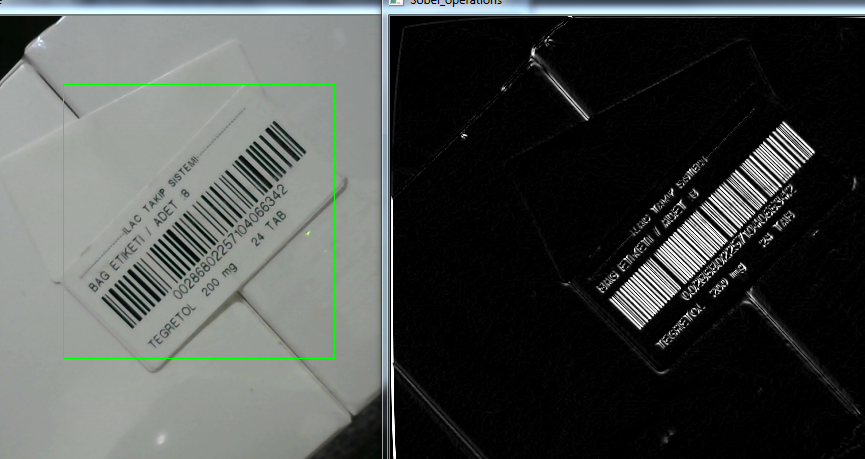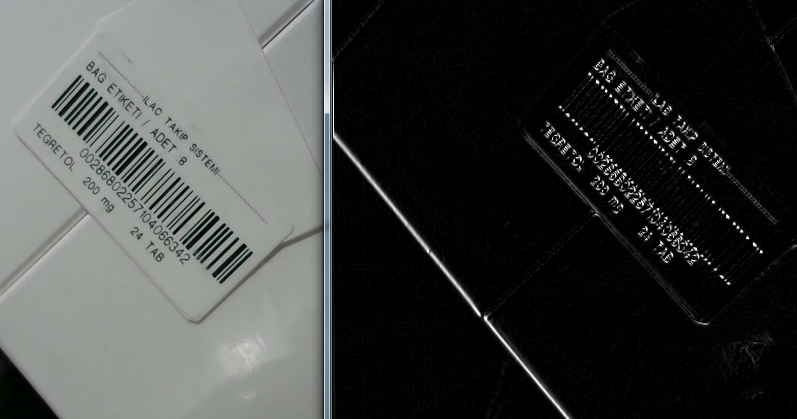Revision history [back]

Barcode recognition, Sobel derivatives and image transformation problem!

First of all, I am developing a program for barcode reading.I use Sobel derivatives to obtain Gradient representation of image in x and y direction for barcode bars.It works very well in both directions(0 to 270 degree).

But unfortunately my sobel derivative barcode can not recognize another angle of image.I don't know how to explain it, but you can understand it by looking at the two different pictures I've added below.

Correctly recognized barcode bars,Can not detect from the same angle in reverse view,Here is my code for sobel derivatives , I have added y direction gradient view to x direction using addweighted command.

//Sobel_variables sobel_variables; vector<sobel_variables> sobel_variables(1); sobel_variables.alpha = 1;

sobel_variables.beta = 0.9;

Sobel(gray_image, sobel_variables.Gradx, sobel_variables.ddepth, 1, 0, 3);
Sobel(gray_image, sobel_variables.Grady, sobel_variables.ddepth, 0, 1, 3);

//sobel_variables.Out_Image.

imshow("Sobel_operations", sobel_variables.Out_Image);
return sobel_variables.Out_Image;

It is really strange.Any help will be apreciated to solve this problem.

Barcode recognition, Sobel derivatives and image transformation problem!

First of all, I am developing a program for barcode reading.I use Sobel derivatives to obtain Gradient representation of image in x and y direction for barcode bars.It works very well in both directions(0 to 270 degree).

But unfortunately my sobel derivative barcode can not recognize another angle of image.I don't know how to explain it, but you can understand it by looking at the two different pictures I've added below.

Correctly recognized barcode bars,Can not detect from the same angle in reverse view,Here is my code for sobel derivatives , I have added y direction gradient view to x direction using addweighted command.

//Sobel_variables sobel_variables; vector<sobel_variables>

vector<Sobel_variables> sobel_variables(1);
sobel_variables.alpha = 1;

1;

sobel_variables.beta = 0.9;

Sobel(gray_image, sobel_variables.Gradx, sobel_variables.ddepth, 1, 0, 3);
Sobel(gray_image, sobel_variables.Grady, sobel_variables.ddepth, 0, 1, 3);

//sobel_variables.Out_Image.

imshow("Sobel_operations", sobel_variables.Out_Image);
return sobel_variables.Out_Image;

It is really strange.Any help will be apreciated to solve this problem.

Barcode recognition, Sobel derivatives and image transformation problem!

First of all, I am developing a program for barcode reading.I use Sobel derivatives to obtain Gradient representation of image in x and y direction for barcode bars.It works very well in both directions(0 to 270 degree).

But unfortunately my sobel Sobel derivative barcode representation can not recognize another angle of image.I don't know how to explain it, but you can understand it by looking at the two different pictures I've added below.

Correctly recognized barcode bars,Can not detect from the same angle in reverse view,Here is my code for sobel derivatives , I have added y direction gradient view to x direction using addweighted command.

vector<Sobel_variables> sobel_variables(1);
sobel_variables.alpha = 1;

sobel_variables.beta = 0.9;

Sobel(gray_image, sobel_variables.Gradx, sobel_variables.ddepth, 1, 0, 3);
Sobel(gray_image, sobel_variables.Grady, sobel_variables.ddepth, 0, 1, 3);

//sobel_variables.Out_Image.

imshow("Sobel_operations", sobel_variables.Out_Image);
return sobel_variables.Out_Image;

It is really strange.Any help will be apreciated to solve this problem.

Barcode recognition, Sobel derivatives and image transformation problem!

First of all, I am developing a program for barcode reading.I use Sobel derivatives to obtain Gradient representation of image in x and y direction for barcode bars.It works very well in both directions(0 to 270 degree).

But unfortunately Sobel derivative representation can not recognize another angle of the image.I don't know how to explain it, but you can understand it by looking at the two different pictures I've added below.

Correctly recognized barcode bars,Can not detect from the same angle in reverse view,Here is my code for sobel derivatives , I have added y direction gradient view to x direction using addweighted command.

vector<Sobel_variables> sobel_variables(1);
sobel_variables.alpha = 1;

sobel_variables.beta = 0.9;

Sobel(gray_image, sobel_variables.Gradx, sobel_variables.ddepth, 1, 0, 3);
Sobel(gray_image, sobel_variables.Grady, sobel_variables.ddepth, 0, 1, 3);